# DFA that recognizes number of 0 is multiple of 3 on input {0,1}

Finite Automata is known to finite state machine which works with transition from one state to another on every input alphabet, as per the defined rules.
In this article, strings that have number of zeros divisible by 3 are acceptable otherwise not acceptable. on the input alphabet ‘0’ and 1′.

• Determine initial state.
• Transition occurs on every input alphabets.
• Determine weather the self loop should apply or not.
• Mark final state.

Designing DFA step by step :

Step-1:
Make initial state “A”then it is the possibility that there would not be any ‘0’ but have only ‘1’ in the string which is acceptable because 0 is divisible by 3.So in this case nay number of 1’s can be present here and for this put self loop of ‘1’ on initial state “A”.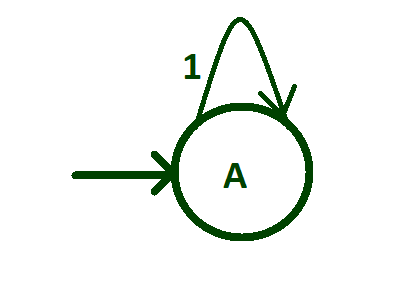Step-2:
Create transition of input alphabet ‘0’ from state “A” to state “B”.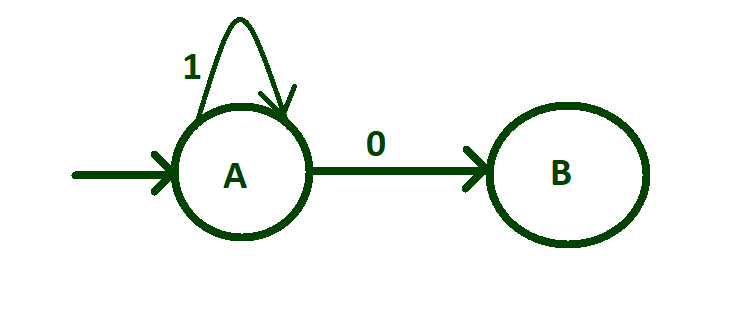Step-3:
After one ‘0’ any number of 1’s can be present i.e, no ‘1’ or more than one ‘1’.For this put self loop of ‘1’ on state “B”.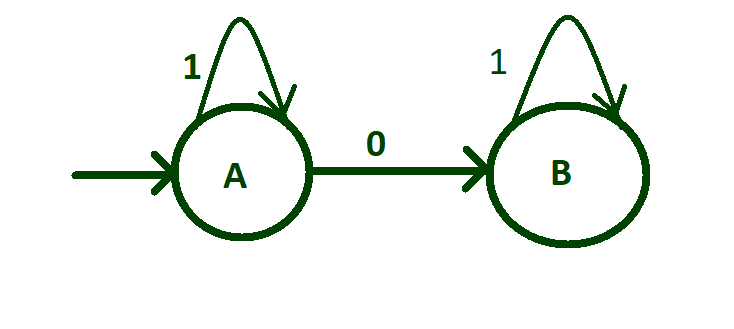Step-4:
Now create transition of input alphabet ‘0’ from state “B” to state “C” and after two 0’s any number of 1’s can be found in the string and for this put self loop of ‘1’ on initial state “C”.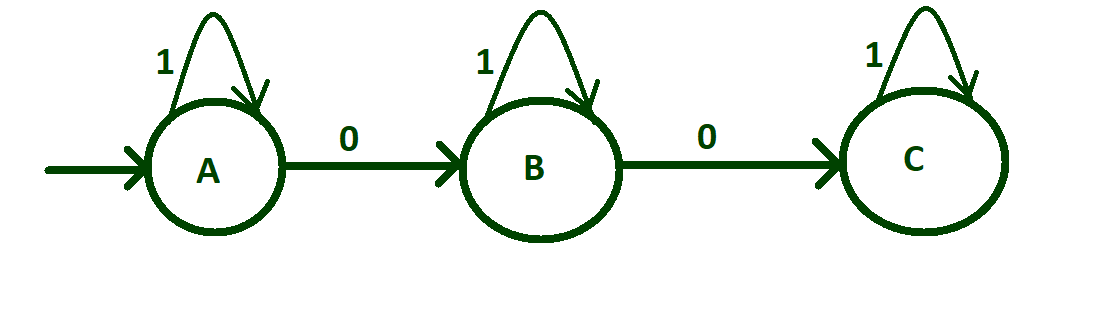Step-5:
Before transition of third ‘0’ we need to think about the logic so that after this transition the machine will accept string that have number of zeros divisible by 3.For this transit ‘o’ from state “C” to state “A”.AS third zero is reaching to state “A” so make state “A” be final state.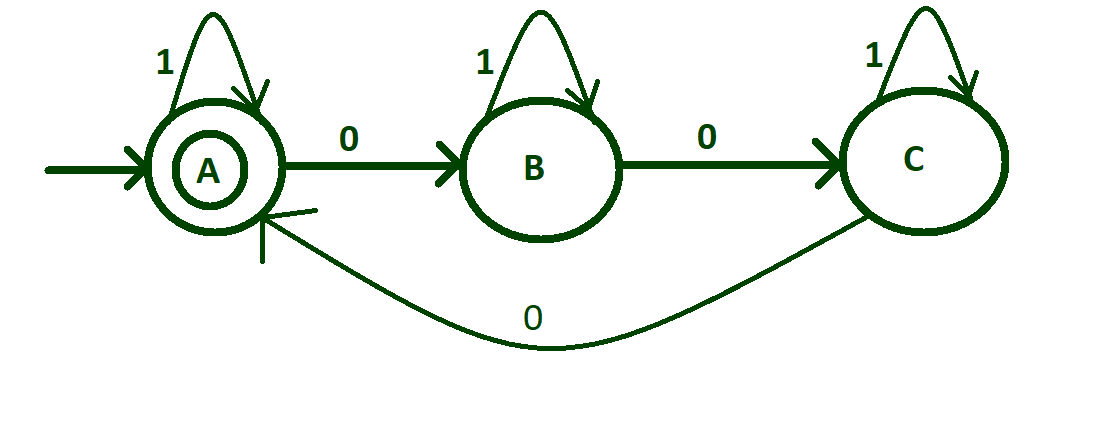Transition table of above DFA:

States Input (0) Input (1)
—> A * B A
B C B
C A C

In above table, —> represents initial state and * represents final state. In this article, initial and final state is same which is final state.

Transition rules of above DFA: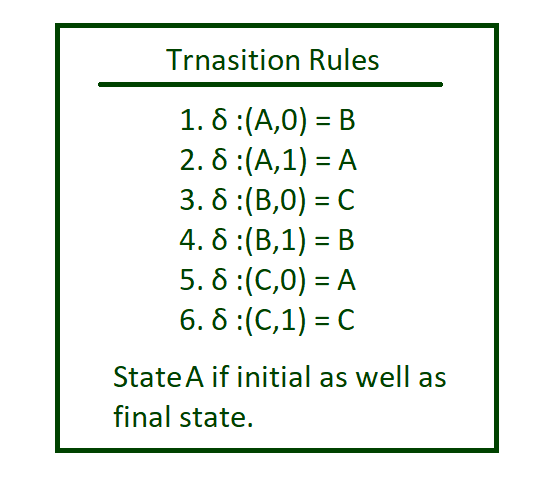Implementing :

## Python3

 `def` `checkStateA(n): ` `     `  `    ``# check length of n  ` `    ``# is 0 then print ` `    ``# string accepted ` `    ``if``(``len``(n)``=``=` `0``): ` `        ``print``(``"string accepted"``) ` `         `  `    ``# if 1 is found call function ` `    ``# checkStateA otherwise if 0 ` `    ``# is found call function stateB ` `    ``else``:     ` `        ``if``(n[``0``]``=``=``'1'``): ` `            ``checkStateA(n[``1``:]) ` `        ``else``: ` `            ``stateB(n[``1``:]) ` `  `  `  `  `def` `stateB(n): ` `     `  `    ``# check length of n  ` `    ``# is 0 then print ` `    ``# string not accepted ` `    ``if``(``len``(n)``=``=` `0``): ` `        ``print``(``"string not accepted"``) ` `         `  `    ``# if 1 is found call function ` `    ``# stateB otherwise if 0 ` `    ``# is found call function stateC     ` `    ``else``:     ` `        ``if``(n[``0``]``=``=``'1'``): ` `            ``stateB(n[``1``:]) ` `        ``else``: ` `            ``stateC(n[``1``:]) ` `        `  `         `  `def` `stateC(n): ` `     `  `    ``# check length of n  ` `    ``# is 0 then print ` `    ``# string not accepted ` `    ``if``(``len``(n)``=``=` `0``): ` `        ``print``(``"string not accepted"``) ` `         `  `    ``# if 1 is found call function ` `    ``# stateC otherwise if 0 ` `    ``# is found call function checkStateA  ` `    ``else``:     ` `        ``if``(n[``0``]``=``=``'1'``): ` `            ``stateC(n[``1``:]) ` `        ``else``: ` `            ``checkStateA(n[``1``:]) ` `         `  `# take string input ` `n ``=` `input``() ` ` `  `# call checkStateA ` `# to check the inputted string ` `checkStateA(n) `

Attention reader! Don’t stop learning now. Get hold of all the important CS Theory concepts for SDE interviews with the CS Theory Course at a student-friendly price and become industry ready.

My Personal Notes arrow_drop_upCheck out this Author's contributed articles.

If you like GeeksforGeeks and would like to contribute, you can also write an article using contribute.geeksforgeeks.org or mail your article to contribute@geeksforgeeks.org. See your article appearing on the GeeksforGeeks main page and help other Geeks.

Please Improve this article if you find anything incorrect by clicking on the "Improve Article" button below.

Article Tags :

1

Please write to us at contribute@geeksforgeeks.org to report any issue with the above content.